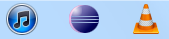# Java solution to Project Euler Problem 12

The sequence of triangle numbers is generated by adding the natural numbers. So the 7th triangle number would be 1 + 2 + 3 + 4 + 5 + 6 + 7 = 28. The first ten terms would be:

1, 3, 6, 10, 15, 21, 28, 36, 45, 55, …

Let us list the factors of the first seven triangle numbers:
01: 1
03: 1,3
06: 1,2,3,6
10: 1,2,5,10
15: 1,3,5,15
21: 1,3,7,21
28: 1,2,4,7,14,28

We can see that 28 is the first triangle number to have over five divisors.

What is the value of the first triangle number to have over five hundred divisors?

Running time: 1325ms (1.3 sec)

Assessment: There’s probably a better/faster way to do this.

```public class Problem012
{
private static int countFactors(int f)
{
int factors = 0;

for (int i = 1; i <= Math.sqrt(f); i++)
{
if (f % i == 0)
factors += 2;
}

return factors;
}

public static void main(String[] args)
{
long begin = System.currentTimeMillis();
int iter = 1;
int size = 1;
int NumFactors = 0;

while (NumFactors <= 500)
{
NumFactors = countFactors(size);
iter++;
size += iter;
}

System.out.println(size-iter);
long end = System.currentTimeMillis();
System.out.println(end-begin + "ms");
}
}```

# Java solution to Project Euler Problem 8

Find the greatest product of five consecutive digits in the 1000-digit number.

73167176531330624919225119674426574742355349194934
96983520312774506326239578318016984801869478851843
85861560789112949495459501737958331952853208805511
12540698747158523863050715693290963295227443043557
66896648950445244523161731856403098711121722383113
62229893423380308135336276614282806444486645238749
30358907296290491560440772390713810515859307960866
70172427121883998797908792274921901699720888093776
65727333001053367881220235421809751254540594752243
52584907711670556013604839586446706324415722155397
53697817977846174064955149290862569321978468622482
83972241375657056057490261407972968652414535100474
82166370484403199890008895243450658541227588666881
16427171479924442928230863465674813919123162824586
17866458359124566529476545682848912883142607690042
24219022671055626321111109370544217506941658960408
07198403850962455444362981230987879927244284909188
84580156166097919133875499200524063689912560717606
05886116467109405077541002256983155200055935729725
71636269561882670428252483600823257530420752963450

Running time: 35ms

Assessment: Pretty straightforward; just iterate through the number character by character and check it.

```public class Problem008
{
public static int checkInt(String s)
{
int product = 1;

for (int i = 0; i < 5; i++)
{
Character c = new Character(s.charAt(i));
String tmp = c.toString();
int temp = Integer.parseInt(tmp);
product *= temp;
}
return product;
}

public static void main(String[] args)
{
long begin = System.currentTimeMillis();
String BigNum = "7316717653133062491922511967442657474235534919493496983520312774506326239578318016984801869478851843858615607891129494954595017379583319528532088055111254069874715852386305071569329096329522744304355766896648950445244523161731856403098711121722383113622298934233803081353362766142828064444866452387493035890729629049156044077239071381051585930796086670172427121883998797908792274921901699720888093776657273330010533678812202354218097512545405947522435258490771167055601360483958644670632441572215539753697817977846174064955149290862569321978468622482839722413756570560574902614079729686524145351004748216637048440319989000889524345065854122758866688116427171479924442928230863465674813919123162824586178664583591245665294765456828489128831426076900422421902267105562632111110937054421750694165896040807198403850962455444362981230987879927244284909188845801561660979191338754992005240636899125607176060588611646710940507754100225698315520005593572972571636269561882670428252483600823257530420752963450";
String snip;
int largest = 0;

for (int i = 0; i <= (BigNum.length()-5); i++)
{
snip = "";

for (int j = 0; j < 5; j++)
{
char c = BigNum.charAt(i+j);
snip += c;
}

if (checkInt(snip) > largest)
largest = checkInt(snip);
}
long end = System.currentTimeMillis();
System.out.println(largest);
System.out.println(end-begin + "ms");
}
}```

# Java solution to Project Euler Problem 7

By listing the first six prime numbers: 2, 3, 5, 7, 11, and 13, we can see that the 6th prime is 13.

What is the 10001st prime number?

Running time: 10010ms (10 sec)

Assessment: This is a classic example of a bad factorization algorithm, because the results aren’t memoized in any way. I promise my solutions get a lot better right around Problem 21.

```public class Problem007
{
private static boolean isPrime(long n)
{
//returns 0 if not prime, 1 if prime
if ((n % 2 == 0)&&(n != 2))
return false;		// is even, therefore not prime
for (long i = 3; i <= (n^(1/2)+1); i += 2)	// Skip all the even numbers
{
if (n % i == 0)
return false;	//not prime
}
return true;
}

public static void main(String[] args)
{
long begin = System.currentTimeMillis();
int NumPrimes = 1;
long i = 2;
while(NumPrimes <= 10001)
{
if (isPrime(i))
{
i++;
NumPrimes++;
}
else i++;
}
long end = System.currentTimeMillis();
System.out.println(i);
System.out.println(end-begin + "ms");
}
}```

# Java solution to Project Euler Problem 6

The sum of the squares of the first ten natural numbers is,
1^2 + 2^2 + … + 10^2 = 385

The square of the sum of the first ten natural numbers is,
(1 + 2 + … + 10)^2 = 55^2 = 3025

Hence the difference between the sum of the squares of the first ten natural numbers and the square of the sum is
3025 – 385 = 2640.

Find the difference between the sum of the squares of the first one hundred natural numbers and the square of the sum.

Running time: 0ms

Assessment: I exhibited symptoms of brain inversion the first time I read the problem description. I was very tired when I wrote this. How do I know? There are zombies in my code.```public class Problem006
{
private static long sum_squares(int n)
{
int total = 0;
for (int i = 1; i <= n; i++)
total += (i*i);
System.out.println("sum_squares() = " + total);
}

private static long squares_sum(int n)
{
long total = 0;
for (int i = 1; i <= n; i++)
total += i;
total *= total;
System.out.println("squares_sum() = " + total);
}

public static void main(String args[])
{
long begin = System.currentTimeMillis();
long omg_zombies = squares_sum(100) - sum_squares(100);
long end = System.currentTimeMillis();
System.out.println(omg_zombies);
System.out.println(end-begin + "ms");
}
}```

# Java solution to Project Euler Problem 5

2520 is the smallest number that can be divided by each of the numbers from 1 to 10 without any remainder.

What is the smallest positive number that is evenly divisible by all of the numbers from 1 to 20?

Running time: 125ms

Assessment: LOL.

```public class Problem005
{
public static void main(String[] args)
{
long begin = System.currentTimeMillis();
int i = 20;
while (true)
{
if (	(i % 1 == 0) &&
(i % 2 == 0) &&
(i % 3 == 0) &&
(i % 4 == 0) &&
(i % 5 == 0) &&
(i % 6 == 0) &&
(i % 7 == 0) &&
(i % 8 == 0) &&
(i % 9 == 0) &&
(i % 10 == 0) &&
(i % 11 == 0) &&
(i % 12 == 0) &&
(i % 13 == 0) &&
(i % 14 == 0) &&
(i % 15 == 0) &&
(i % 16 == 0) &&
(i % 17 == 0) &&
(i % 18 == 0) &&
(i % 19 == 0) &&
(i % 20 == 0) )
{
break;
}
i += 20;
}
long end = System.currentTimeMillis();
System.out.println(i);
System.out.println(end-begin + "ms");
}
}```

# Java solution to Project Euler Problem 4

A palindromic number reads the same both ways. The largest palindrome made from the product of two 2-digit numbers is 9009 = 91 99.

Find the largest palindrome made from the product of two 3-digit numbers.

Running time: 5ms

Assessment: Despite finishing quickly, this is a bad solution, because it returns the first time it reaches a palindrome. The problem is that there’s no guarantee that the return value is, in fact, the correct answer. Using different input criteria could easily break it. It so happens that the returned value of this code is correct, but this was more luck than skill.

```public class Problem004
{
private static int createPalindrome()
{
int total = 0;
for (int i = 999; i >= 900; i--)
{
for (int j = 999; j >= 900; j--)
{
total = i * j;
String s = Integer.toString(total);
String s2 = new StringBuffer(s).reverse().toString();
if (s.equals(s2))
}
}
return 0;
}

public static void main(String[] args)
{
long begin = System.currentTimeMillis();
long end = System.currentTimeMillis();
System.out.println(end-begin + "ms");
}
}```

# Java solution to Project Euler Problem 3

The prime factors of 13195 are 5, 7, 13 and 29.

What is the largest prime factor of the number 600851475143 ?

Running time: 2ms

Assessment: This was when I switched from C++ to Java, and this solution is a lot better than my C++ solution. This one finishes, and finishes pretty quickly.

```public class Problem003b
{
public static void main(String[] args)
{
long begin = System.currentTimeMillis();
long n=600851475143L;
for (long i = 2; i <= n; i++)
{
if (n % i==0)
{
System.out.println(i);
n = n / i;
i = 2;
}
}
long end = System.currentTimeMillis();
System.out.println(end-begin + "ms");
}
}```

# How-to: Pin Eclipse to the Windows 7 taskbar

Before you begin, close any and all instances of Eclipse.

The Eclipse IDE for Java doesn’t come with an installer like most Windows packages do; it’s a zip file containing the program and the libraries it needs to run. As a result of this, and the fact that it’s written in Java, it has some non-standard behavior characteristics on its edges. One of them is the inability to pin the program to the taskbar like you can with most Windows programs. Another is the fact that when you tap the Windows key and type “eclipse”, eclipse.exe often doesn’t show up. (It might if you put it someplace besides %ProgramFiles% or %ProgramFiles(x86)%, I haven’t tested.)I use the Windows 7 taskbar heavily, because I like being able to launch programs using Win+[number]. My desktop and laptop are configured identically, so Win+1 always opens Chrome, Win+5 is always iTunes. If you launch eclipse using Windows explorer, and then you try to “pin” the program to the taskbar, you discover that you can’t: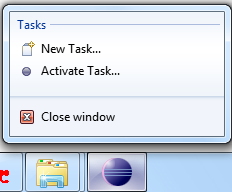Well there’s a workaround. Buried in this bug report, you’ll discover that if you edit your environment variables, you’ll be able to pin eclipse to the taskbar. (You’ll need to be logged in as an Administrator):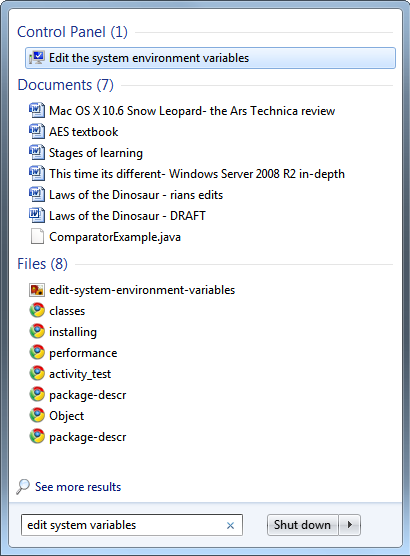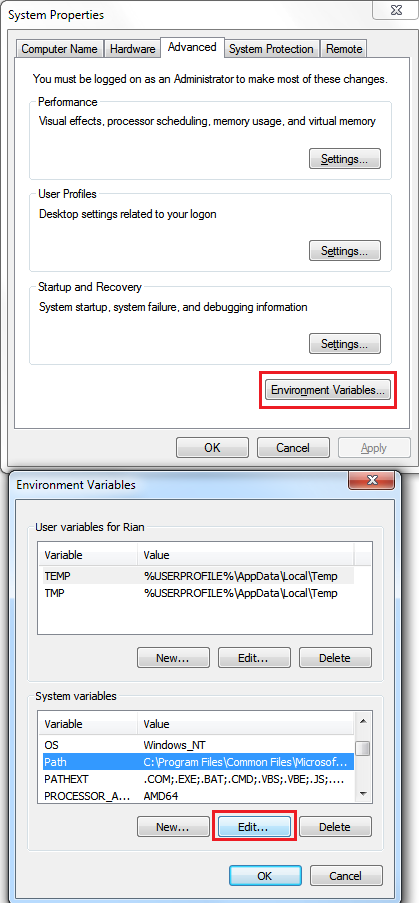Add the path to the JRE to what’s currently there, followed by a semicolon. In my case, that would be:

`C:\Program Files\Java\jre6\bin;`

While you’re editing environment variables, it might not hurt to put in the path to the JDK, so if you ever decide to do Java development outside Eclipse, you don’t need to resort to setting the path by hand every time you launch the CLI. For me that’s “C:\Program Files\Java\jdk1.6.0_22\bin;” but it will change as newer versions of Java are released. If you do this, make sure you put the JDK path after the JRE path. For non-development purposes, you’ll generally want to be using the latest version of the JRE.

My new path looks like this:

`C:\Program Files\Java\jre6\bin;C:\Program Files\Java\jdk1.6.0_22\bin;C:\Program Files\Common Files\Microsoft Shared\Windows Live;[etc]`

Click OK, and launch Eclipse from explorer as you normally would. You should now be able to pin Eclipse to the taskbar, giving it a home among the rest of your permanently docked programs. Doing it this way means you won’t see two icons when Eclipse is open, which is what happens if you drag eclipse.exe to the taskbar without editing the system path.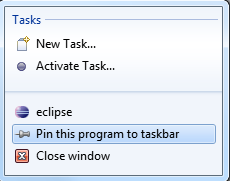Gives you: